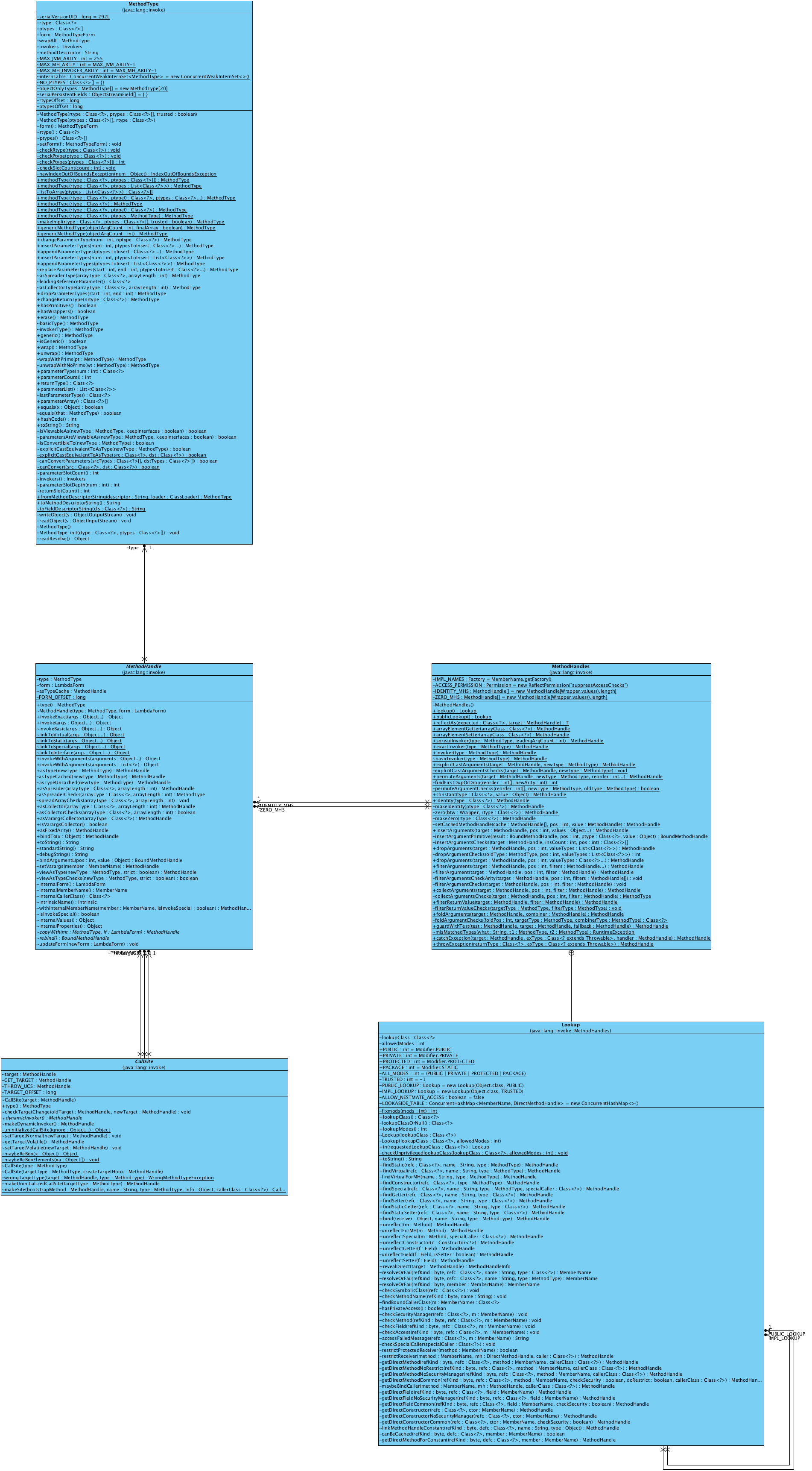## Java 8 里面的双冒号语法和lambda一样，也是通过invokedynamic来实现的

Java8里面引入了下面这种双冒号的语法形式：

``````import java.util.function.IntBinaryOperator;

public class DoubleColon {
public static int testWith(IntBinaryOperator op, int left, int right) {
return op.applyAsInt(left, right);
}

public static void main(String[] args) throws Exception {
System.out.println(testWith(Math::max, 1, 2));
}
}
``````

``````package java.util.function;

/**
* Represents an operation upon two {@code int}-valued operands and producing an
* {@code int}-valued result.   This is the primitive type specialization of
* {@link BinaryOperator} for {@code int}.
*
* <p>This is a <a href="package-summary.html">functional interface</a>
* whose functional method is {@link #applyAsInt(int, int)}.
*
* @see BinaryOperator
* @see IntUnaryOperator
* @since 1.8
*/
@FunctionalInterface
public interface IntBinaryOperator {

/**
* Applies this operator to the given operands.
*
* @param left the first operand
* @param right the second operand
* @return the operator result
*/
int applyAsInt(int left, int right);
}
``````

``````
import java.util.function.IntBinaryOperator;

public class IntBinaryOperatorImpl implements IntBinaryOperator {
@Override
public int applyAsInt(int left, int right) {
return Math.max(left, right);
}
}
``````

``````testWith(new IntBinaryOperatorImpl());
``````

``````testWith(Math::max);
````````````import java.lang.invoke.MethodHandle;
import java.lang.invoke.MethodHandles;
import java.lang.invoke.MethodType;

public class MethodHandleDemo {
public static void main(String[] args) throws Throwable {
MethodHandles.Lookup lookup = MethodHandles.lookup();
MethodHandle handle =
lookup.findStatic(MethodHandleDemo.class, "hello", MethodType.methodType(void.class));
handle.invokeExact();
}

static void hello() {
System.out.println("Hello");
}
}
``````

P.S.

`InvokeDynamic 101`1这篇文章里，作者提供了一个完整的，使用`invokedynamic`指令的例子。首先是`MHD` class：

``````import java.lang.invoke.MethodHandle;
import java.lang.invoke.MethodHandles;
import java.lang.invoke.MethodType;

public class MHD {
public static void main(String[] args) throws Throwable {
MethodHandles.Lookup lookup = MethodHandles.lookup();
MethodHandle handle = lookup.findStatic(Math.class, "pow",
MethodType.methodType(double.class,
double.class,
double.class));
handle = MethodHandles.insertArguments(handle, 1, 10);
System.out.printf("2^10 = %d%n", (int) (double) handle.invoke(2.0));
}
}
``````

1. http://www.javaworld.com/article/2860079/learn-java/invokedynamic-101.html?page=2